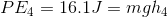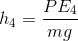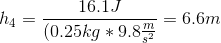# AP Physics B : Understanding Conservation of Energy

## Example Questions

### Example Question #1 : Understanding Conservation Of Energy

A ball is thrown upwards with a velocity of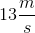. Use conservation of energy to find the ball's maximum height.

Not enough information to solve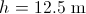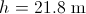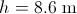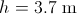Explanation:

We could approach this problem by using one of the kinematics equations but we should use conservation of energy to solve. Conservation of energy states that the initial total energy of a system is equal to its final total energy. In our case, the system is a rock with an initial speed of. Choosing the instant at which the rock was thrown at zero height, the rock has only has kinetic energy initially.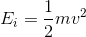At maximum height, the velocity is zero (no kinetic energy) and the ball will have only potential energy.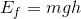By conservation of energy, we know that the initial and final energies are equal.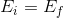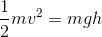Using this, we can solve for the height.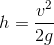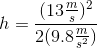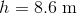### Example Question #2 : Understanding Conservation Of Energy

A ball is thrown upward and reaches a maximum height of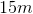. What was the initial velocity of the ball?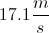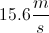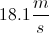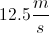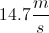Explanation:

This question can be solved using either conservation of energy or linear motion equations.

The ball reaches maximum height when all of the initial kinetic energy is converted to potential energy. From this, we use the relationship: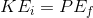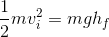Mass cancels out from the equation, and we can isolate the velocity variable.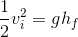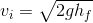We are given the final height and the acceleration from gravity, allowing us to find the initial velocity.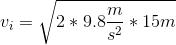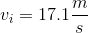### Example Question #51 : Ap Physics B

A rock is dropped from rest from a height of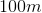. What is the velocity of the rock right before it hits the ground?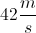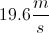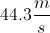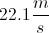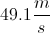Explanation:

We can use conservation of energy to solve this problem. The rock initially has a potential energy, but no kinetic energy. When the rock reaches the ground, it has no potential energy and all kinetic energy. By conservation of energy: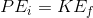Substitute the equations for potential and kinetic energy.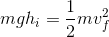We can cancel the mass from each term, and isolate the final velocity variable.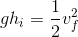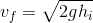Finally, use the given value for the height and the acceleration of gravity to solve for the final velocity.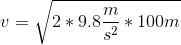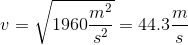### Example Question #4 : Understanding Conservation Of Energyblock falls vertically from rest, under the influence of only a vertical gravitational field, onto a long vertical spring with spring constant of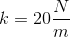. It compresses the spring directly downward. If the block is moving at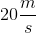at the moment it contacts the spring, what is the total vertical distance it travels from the release point until the instant it comes to rest at maximum spring compression?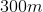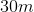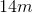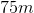Explanation:

Take the upper end of the spring as “zero.” Since both the spring force and gravitation are conservative forces, the total energy of the block will be conserved as it falls. Knowing the velocity at the upper end of the spring allows calculation of the block’s kinetic energy at that point.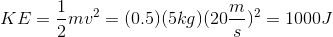The kinetic energy equals the potential energy it has at its maximum height; since it is falling from rest, it has no kinetic energy at the start. The kinetic energy also equals the maximum spring potential energy the block has at maximum spring compression (where the block has once again come momentarily to rest, and thus has zero kinetic energy).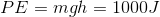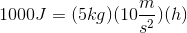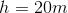Now we will need to look at the spring potential energy.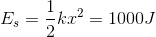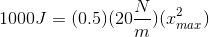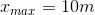So the total vertical distance traveled is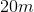of fall with an additional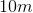of spring compression for a final distance of.

### Example Question #5 : Understanding Conservation Of Energy

Scientists invent a frictionless surface that allows any object to slide without losing energy to friction. How would the energy of a ball change if it were rolled down the side of a bowl made of this frictionless material in a vacuum?

Only kinetic energy would change

Potential and kinetic energy would both gradually decrease and eventually become zero

Both kinetic and potential energy would constantly change

There would be no change in energy

Internal energy would decrease when the ball rolls upward

Both kinetic and potential energy would constantly change

Explanation:

The bowl described is, fundamentally, a perpetual motion device. When a ball is rolled down the side of a bowl it will gather kinetic energy. As it rolls up the opposite side, this kinetic energy is converted to potential energy until the ball reaches a maximum height. At this point, potential is converted back to kinetic energy. This process would repeat infinitely as long as no energy is lost to the outside environment.

In reality, this machine cannot exist. While a frictionless surface would prevent loss of energy as heat and the vacuum would prevent air resistance, these conditions cannot be met in the real world.

In the given scenario, however, total energy never decreases. The maximum potential energy will always be the same, meaning that the ball will always reach the same maximum height, and the maximum kinetic energy will always be the same, meaning that the ball will always reach the same maximum velocity. Since no energy is lost, both kinetic and potential energy will constantly change in relation to one another, but total mechanical energy will remain forever constant.

### Example Question #6 : Understanding Conservation Of Energy

A ball is fired directly upward and has a flight time of six seconds. At what time in the trajectory will the kinetic energy and potential energy of the ball be equal?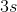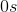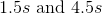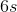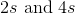Explanation:

This question can be solved by using conservation of energy. Initially, the ball has a displacement of zero, but a non-zero initial velocity. We can say that the initial energy is equal to the total kinetic energy.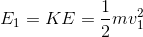At the peak height of the trajectory, the velocity will be zero and the ball will have a maximum displacement. We can say that the energy at this point is equal to the total potential energy.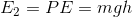Finally, when the ball lands, its displacement will again be zero and it will have accelerated (due to gravity) to regain the same magnitude of velocity as the initial throw.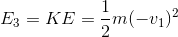Due to conservation of energy, these terms are all equal. As potential energy increases, kinetic energy decreases, and vice versa. To solve this question, we need to know when the potential energy is equal to half of the total energy.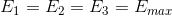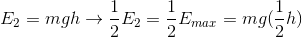At exactly half the height, the potential energy will comprise exactly half the total energy. By necessity, the other half will be kinetic energy and the two values will be equal.

The time to travel half the height will be equal to the full flight time divided by four, since acceleration remains constant. The ball will be at this height twice during its trajectory: when it is traveling upward and when it is traveling down. The second time point will be equal to three times this interval.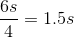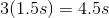At these two times, the kinetic energy and potential energy will have equal values.

### Example Question #1 : Understanding Conservation Of Energy

A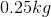rubber ball is released from a height ofand is allowed to bounce repeatedly. Each time the ball strikes the ground, ten percent of the ball's kinetic energy is lost in the collision. What is the maximum height the ball will reach after hitting the ground four times?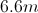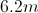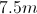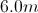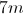Explanation:

Each time the ball bounces, 10% of the kinetic energy is lost. This means that after colliding with the ground, the ball has 90% of its previous potential energy at the top of its flight. To find the total energy after four bounces, we multiply the initial potential energy by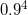.

The initial potential energy is: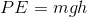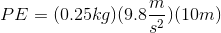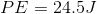Use our exponential expression to find the remaining energy after four bounces.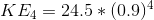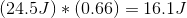Based on conservation of energy, we can find the height that the ball travels with this remaining kinetic energy